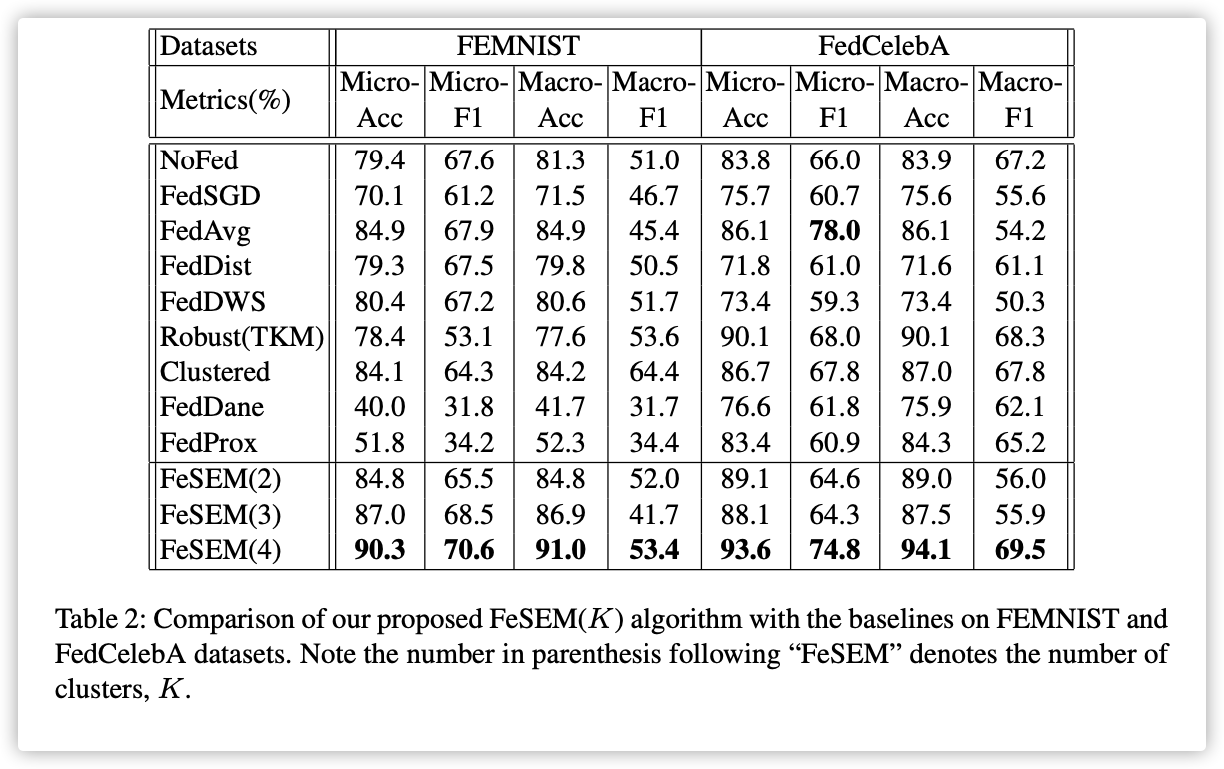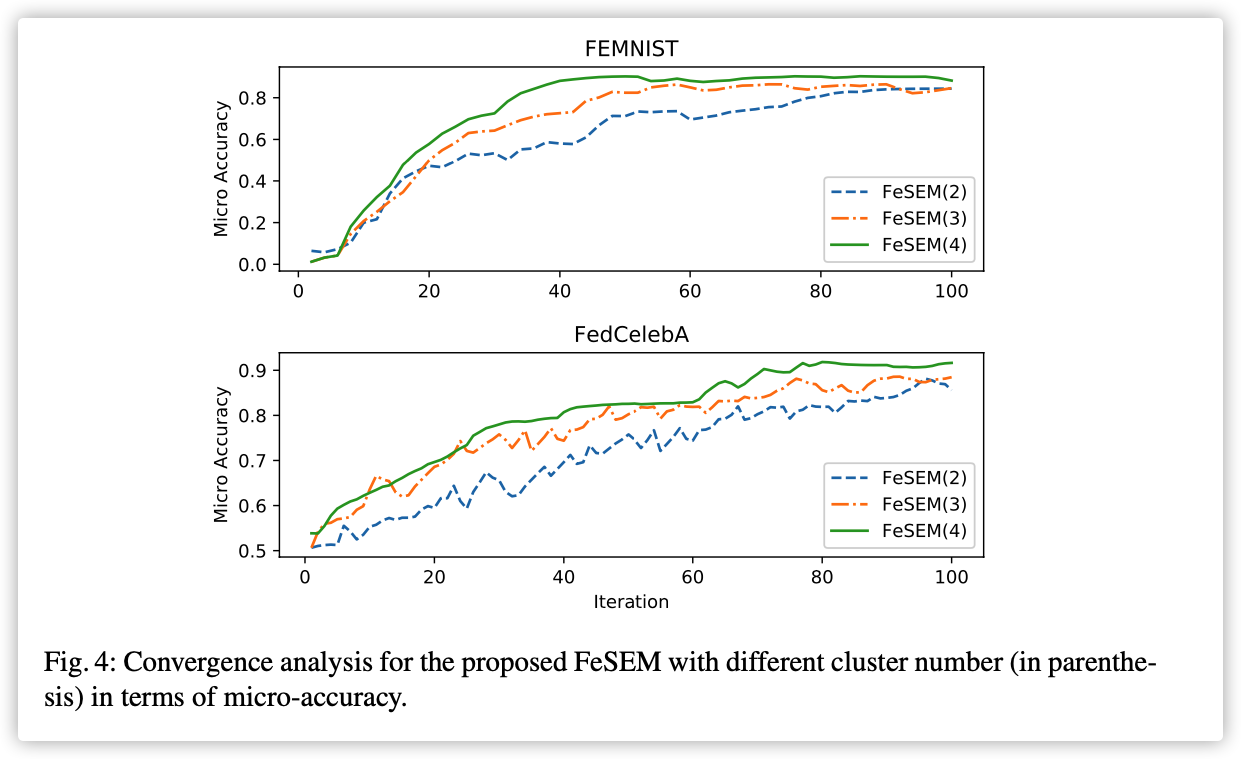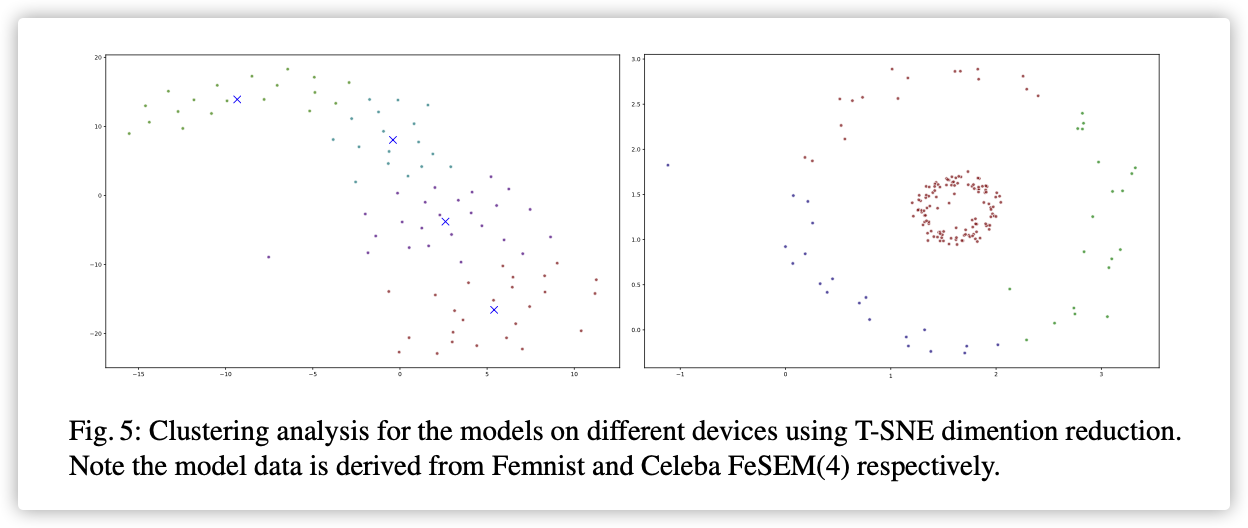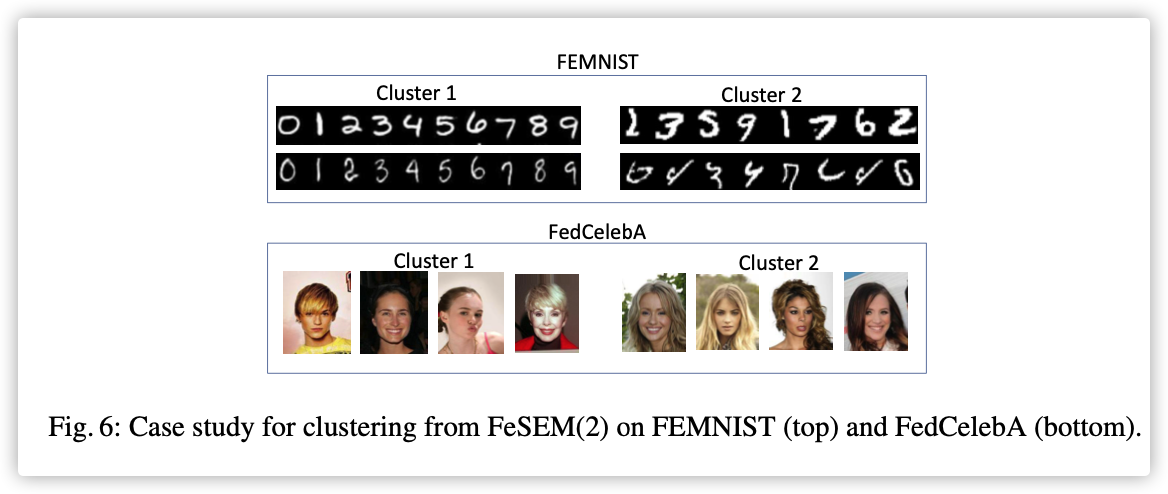## 0x00 Abstract

Existing federated learning approaches usually adopt a single global model to capture the shared knowledge of all users by aggregating their gradients, regardless of the discrepancy between their data distributions.

Our paper proposes a novel multi-center aggregation mechanism for federated learning, which learns multiple global models from the non-IID user data and simultaneously derives the optimal matching between users and centers.

We formulate the problem as a joint optimization that can be efficiently solved by a stochastic expectation maximization (EM) algorithm.

## 0x01 Introduction

Early federated learning approaches use only one global model as a single-center to aggregate the information of all users. The stochastic gradient descent (SGD) for single-center aggregation is designed for IID data, and therefore, conflicts with the non-IID setting in federated learning.

Sattler.F proposed an idea of clustered federated learning (FedCluster) that addresses the non-IID issue by dividing the users into multiple clusters. However, the hierarchical clustering in FedCluster is achieved by multiple rounds of bipartite separation, each requiring to run the federated SGD algorithm till convergence.

We formulate the problem of multi-center federated learning as jointly optimizing the clustering of users and the global model for each cluster such that 1) each user’s local model is assigned to its closest global model, and 2) each global model leads to the smallest loss over all the users in the associated cluster.

Main contribution:

• We propose a novel multi-center aggregation approach (Section 4.1) to address the non-IID challenge of federated learning.
• We design an objective function, namely multi-center federated loss (Section 4.2), for user clustering in our problem.
• We propose Federated Stochastic Expectation Maximization (FeSEM) (Section4.3) to solve the optimization of the proposed objective function.
• We present the algorithm (Section 4.4) as an easy-to-implement and strong baseline for federated learning . Its effectiveness is evaluated on benchmark datasets. (Section 5)

To solve the problem caused by non-IID or heterogeneity data in federated setting:

"Clustered federated learning: Model-agnostic distributed multi-task optimization under privacy constraints" proposed clustered federated learning (FedCluster) by integrating federated learning and bi-partitioning-based clustering into an overall framework.

“Robust federated learning in a heterogeneous environment” proposed robust federated learning composed of three steps: 1) learning a local model on each device, 2) clustering model parameters to multiple groups, each being a homogeneous dataset, and 3) running a robust distributed optimization in each cluster.

“Feddane: A federated newton-type method” proposed FedDANE by adapting the DANE to federated setting. In particular, FedDANE is a federated Newton-Type optimization method.

“Federated optimization in heterogeneous networks” proposed FedProx for a generalization and re-parameterization of FedAvg. It adds a proximal term to the objective function of each device’s supervised learning task, and the proximal term is to measure the parameter-based distance between the server and the local model.

“Federated learning with personalization layers” added a personalized layer for each local model, i.e., FedPer, to tackle heterogeneous data.

## 0x03 Background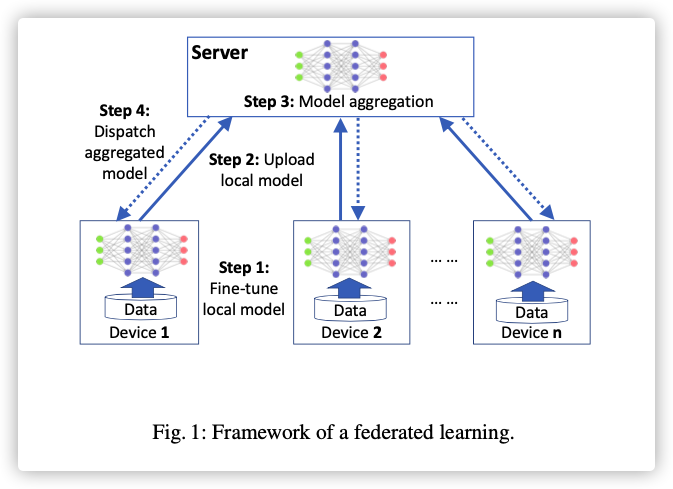• 在拥有隐私数据$\mathcal{D_i}=\{\mathcal{X_i,Y_i}\}$的终端进行监督学习，获得模型$\mathcal{M_i:X_i→Y_i}$

$W_i^{'}=\mathop{argmin}\limits_{W_i}L(\mathcal{M_i,D_i},W_i)$

$L(\cdot)$是损失函数，$W_i$是模型权重。

• 联邦学习考虑到将所有设备的损失函数最小化，根据数据量的多少引入损失的权重,$m$是设备数：

$\mathcal{L}=\sum_{i=1}^m\frac{|\mathcal{D_i}|}{\sum_j|\mathcal{D_j}|}L(\mathcal{M_i,D_i},W_i)$

联邦聚合过程改变如下:

$\tilde{W}^g=\sum_{i=1}^m\frac{|\mathcal{D_i}|}{\sum_j|\mathcal{D_j}|}W_i$

## 0x04 Methodology

### 1.Multi-center Aggregation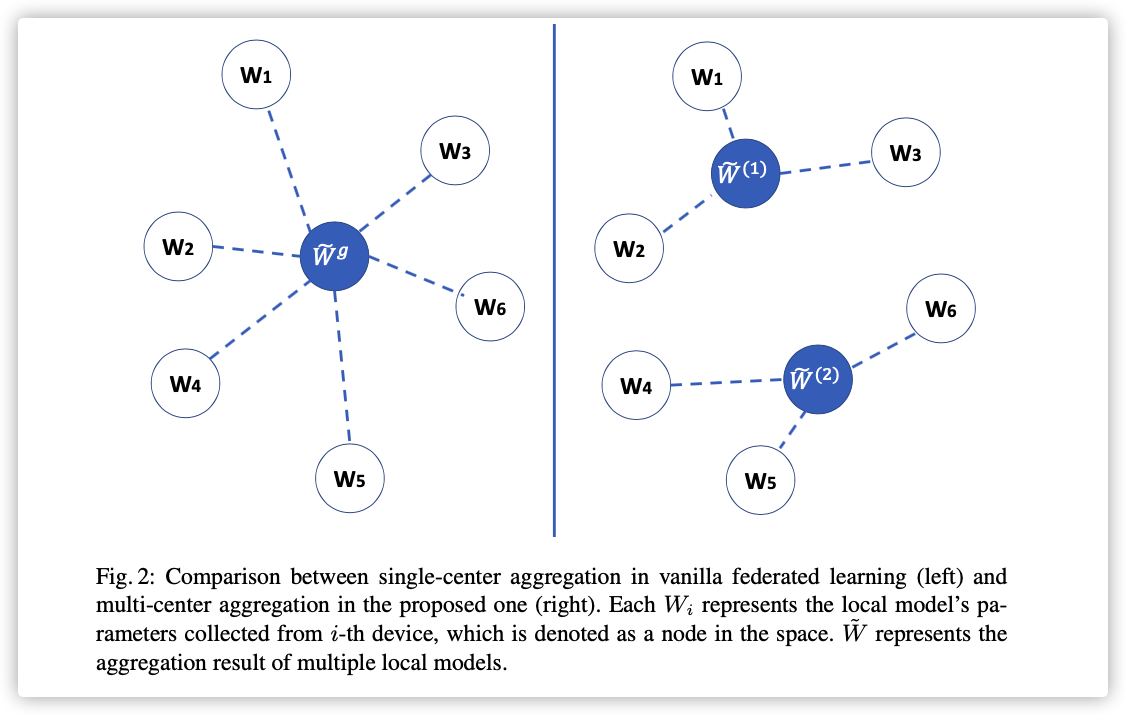### 2.Objective Function

1. distance-based federated loss – a new objective function using a distance between parameters from the global and local models

2. multi-center federated loss – the total distance-based loss to aggregate local models to multiple centers.

#### Distance-based federated loss（DF-Loss）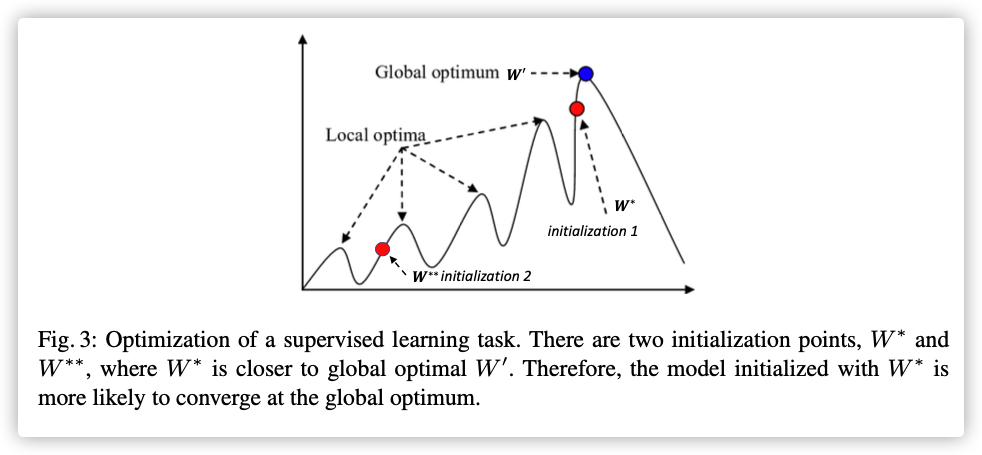Moreover, considering the limited computation power and insufficient training data on each device, a “good” initialization is vitally important to train a supervised learning model on the device.

$DF-Loss$代替原始损失函数的准确性：

“较好”初始模型越靠近$W^*$→越容易收敛到$W^*$→传统损失函数值会降低。

$\mathcal{L}=\frac{1}{m}\sum_{i=1}^mDist(W_i,\tilde{W})$

#### Multi-center DF-Loss

Furthermore, according to the non-IID assumption, the datasets in different devices can be grouped into multiple clusters where the on-device datasets in the same cluster are likely to be generated from one distribution.

$\mathcal{L}=\frac{1}{m}\sum_{k=1}^{K}\sum_{i=1}^m r_i^{(k)}Dist(W_i,\tilde{W}^{(k)})$

### 3.OPtimization Method

• E-step – updating cluster assignment $r_i^{(k)}$ with fixed $W_i$
• M-step – updating cluster centers $\tilde{W}^{(k)}$
• updating local models by providing new initialization $\tilde{W}^{(k)}$.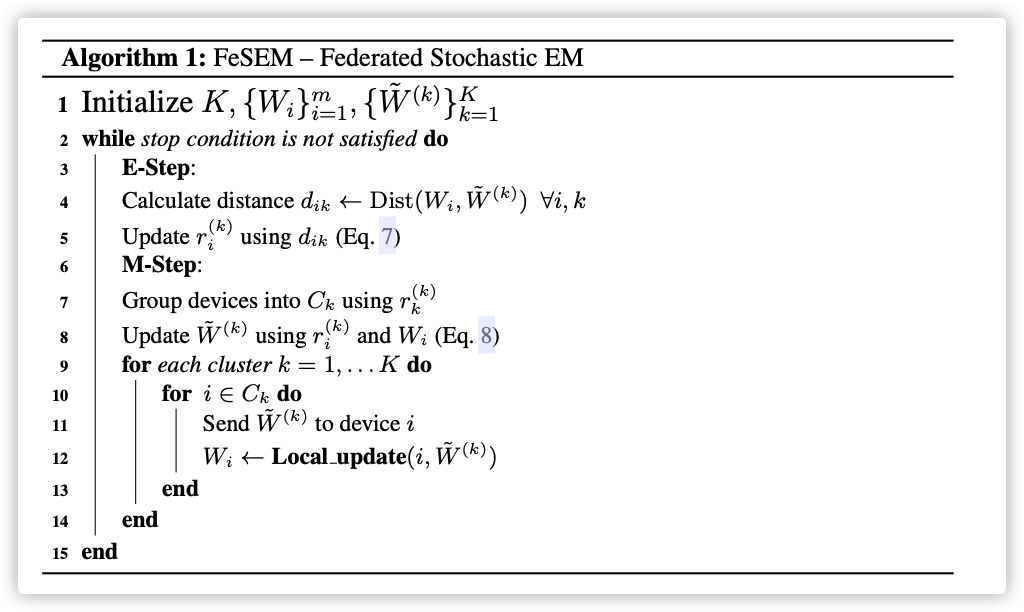E-Step:

\begin{aligned} r_i^{(k)}=\left\{ \begin{array}{l} 1,if k=argmin_jDist(W_i,\tilde{W}^{(j)})\\ 0,otherwise \end{array} \right. \end{aligned}

M-Step:

$\tilde{W}^{(k)}=\frac{1}{\sum^m_{i=1}r_i^{(k)}}\sum_{i=1}^mr_i^{(k)}W_i$

updating the local models:

the global model’s parameters $\tilde{W}^{(k)}$ are sent to each device in cluster $k$ to update its local model, and then we can fine-tune the local model’s parameters $W_i$ using a supervised learning algorithm on its own private training data.

### 4. Algorithm

In particular, in the third step for updating the local model, we need to fine-tune the local model by implementing Algorithm 2.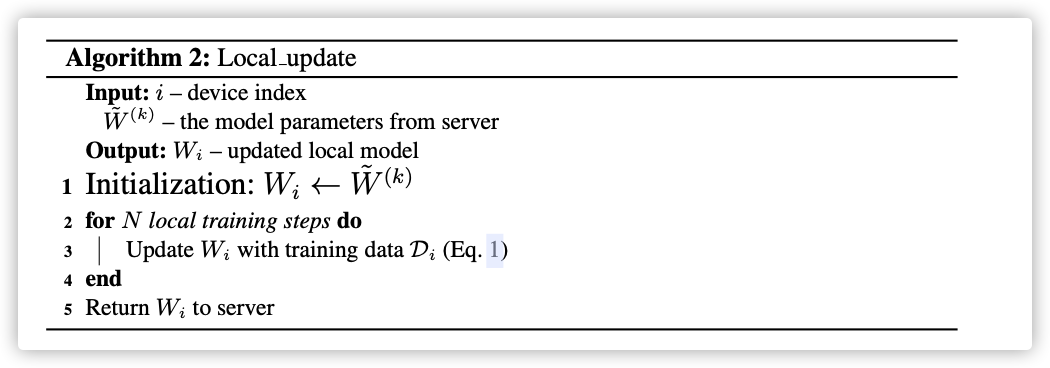## 0x05 Experiments